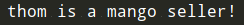# 《算法图解》学习笔记（六）：图和广度优先搜索（附代码）

## 一、图简介1. 使用图来建立问题模型。
2. 使用广度优先搜索解决问题。

## 二、图是什么Alex 欠 Rama 钱，Tom 欠 Adit 钱，等等。图由节点（node）和边（edge）组成。## 三、广度优先搜索

• 第一类问题：从节点A出发，有前往节点B的路径吗？
• 第二类问题：从节点A出发，前往节点B的哪条路径最短？

• 编写国际跳棋AI，计算最少走多少步就可获胜；
• 根据你的人际关系网络找到关系最近的医生。### 1）查找最短路径

• 第一类问题：从节点A出发，有前往节点B的路径吗？（在你的人际关系网中，有芒果销售商吗？）
• 第二类问题：从节点A出发，前往节点B的哪条路径最短？（哪个芒果销售商与你的关系最近？）### 2）队列## 四、实现图graph = {}
graph["you"] = ["alice", "bob", "claire"]graph = {}
graph["you"] = ["alice", "bob", "claire"]
graph["bob"] = ["anuj", "peggy"]
graph["alice"] = ["peggy"]
graph["claire"] = ["thom", "jonny"]
graph["anuj"] = []
graph["peggy"] = []
graph["thom"] = []
graph["jonny"] = []


graph["claire"] = ["thom", "jonny"]
graph["anuj"] = []


graph["anuj"] = []
graph["claire"] = ["thom", "jonny"]


Anuj、Peggy、Thom 和 Jonny 都没有邻居，这是因为虽然有指向他们的箭头，但没有从他们出发指向其他人的箭头。这被称为 有向图（directed graph），其中的关系是单向的。因此，Anuj 是 Bob 的邻居，但 Bob 不是 Anuj 的邻居。无向图（undirected graph） 没有箭头，直接相连的节点互为邻居。例如，下面两个图是等价的。## 五、实现算法from collections import deque
search_queue = deque() # 创建一个队列
search_queue += graph["you"] # 将你的邻居都加入到这个搜索队列中while search_queue: # 只要队列不为空，
person = search_queue.popleft() # 就取出其中的第一个人
if person_is_seller(person): # 检查这个人是否是芒果销售商
print(person + " is a mango seller!") # 是芒果销售商
return True
else:
search_queue += graph[person] # 不是芒果销售商。将这个人的朋友都加入搜索队列
return False # 如果到达了这里，就说明队列中没人是芒果销售商


def person_is_seller(name):
return name[-1] == 'm'• 找到一位芒果销售商；
• 队列变成空的，这意味着你的人际关系网中没有芒果销售商。

Peggy 既是 Alice 的朋友又是 Bob 的朋友，因此她将被加入队列两次：一次是在添加 Alice 的朋友时，另一次是在添加 Bob 的朋友时。因此，搜索队列将包含两个 Peggy。def search(name):
search_queue = deque()
search_queue += graph[name]
searched = [] # 这个数组用于记录检查过的人
while search_queue:
person = search_queue.popleft()
if not person in searched: # 仅当这个人没检查过时才检查
if person_is_seller(person):
print person + " is a mango seller!"
return True
else:
search_queue += graph[person]
searched.append(person) # 将这个人标记为检查过
return False

search("you")


python版本代码如下：

from collections import deque

def person_is_seller(name):
return name[-1] == 'm'

graph = {}
graph["you"] = ["alice", "bob", "claire"]
graph["bob"] = ["anuj", "peggy"]
graph["alice"] = ["peggy"]
graph["claire"] = ["thom", "jonny"]
graph["anuj"] = []
graph["peggy"] = []
graph["thom"] = []
graph["jonny"] = []

def search(name):
search_queue = deque()
search_queue += graph[name]
#这个数组是如何跟踪你以前搜索过的人的
searched = []
while search_queue:
person = search_queue.popleft()
#只有在你还没搜过这个人的时候才搜
if person not in searched:
if person_is_seller(person):
print(person + " is a mango seller!")
return True
else:
search_queue += graph[person]
#将此人标记为已搜索
searched.append(person)
return False

search("you")c++版本代码如下：

#include <iostream>
#include <unordered_map>
#include <string>
#include <vector>
#include <queue>
#include <unordered_set>

using std::cout;
using std::endl;

bool is_seller(const std::string& name) {
return name.back() == 'm';
}

template <typename T>
bool search(const T& name, const std::unordered_map<T, std::vector<T>>& graph) {
std::queue<T> search_queue;
std::unordered_set<T> searched;

// add all friends to search queue
for (auto friend_name : graph.find(name) -> second) {
search_queue.push(friend_name);
}

while (!search_queue.empty()) {
T& person = search_queue.front();
search_queue.pop();

// only search this person if you haven't already searched them.
if (searched.find(person) == searched.end()) {
if (is_seller(person)) {
cout << person << " is a mango seller!" << endl;
return true;
}
std::vector<T> friend_list = graph.find(person) -> second;

// add all friends of a person to search queue
for (T friend_name : friend_list) {
search_queue.push(friend_name);
}

// mark this person as searched
searched.insert(person);
}
}

return false;
}

int main() {
std::unordered_map<std::string, std::vector<std::string>> graph;
graph.insert({"you", {"alice", "bob", "claire"}});
graph.insert({"bob", {"anuj", "peggy"}});
graph.insert({"alice", {"peggy"}});
graph.insert({"claire", {"thom", "jonny"}});
graph.insert({"anuj", {}});
graph.insert({"peggy", {}});
graph.insert({"thom", {}});
graph.insert({"jonny", {}});

std::string name = "you";
bool result = search(name, graph);
cout << "Found mango seller: " << result << endl;
}


## 七、小结

• 广度优先搜索指出是否有从A到B的路径。
• 如果有，广度优先搜索将找出最短路径。
• 面临类似于寻找最短路径的问题时，可尝试使用图来建立模型，再使用广度优先搜索来解决问题。
• 无向图中的边不带箭头，其中的关系是双向的，例如，ross - rachel表示“ross与rachel约会，而rachel也与ross约会”。
• 队列是先进先出（FIFO）的。
• 栈是后进先出（LIFO）的。
• 你需要按加入顺序检查搜索列表中的人，否则找到的就不是最短路径，因此搜索列表必须是队列。
• 对于检查过的人，务必不要再去检查，否则可能导致无限循环。

## 参考文章

• 《算法图解》